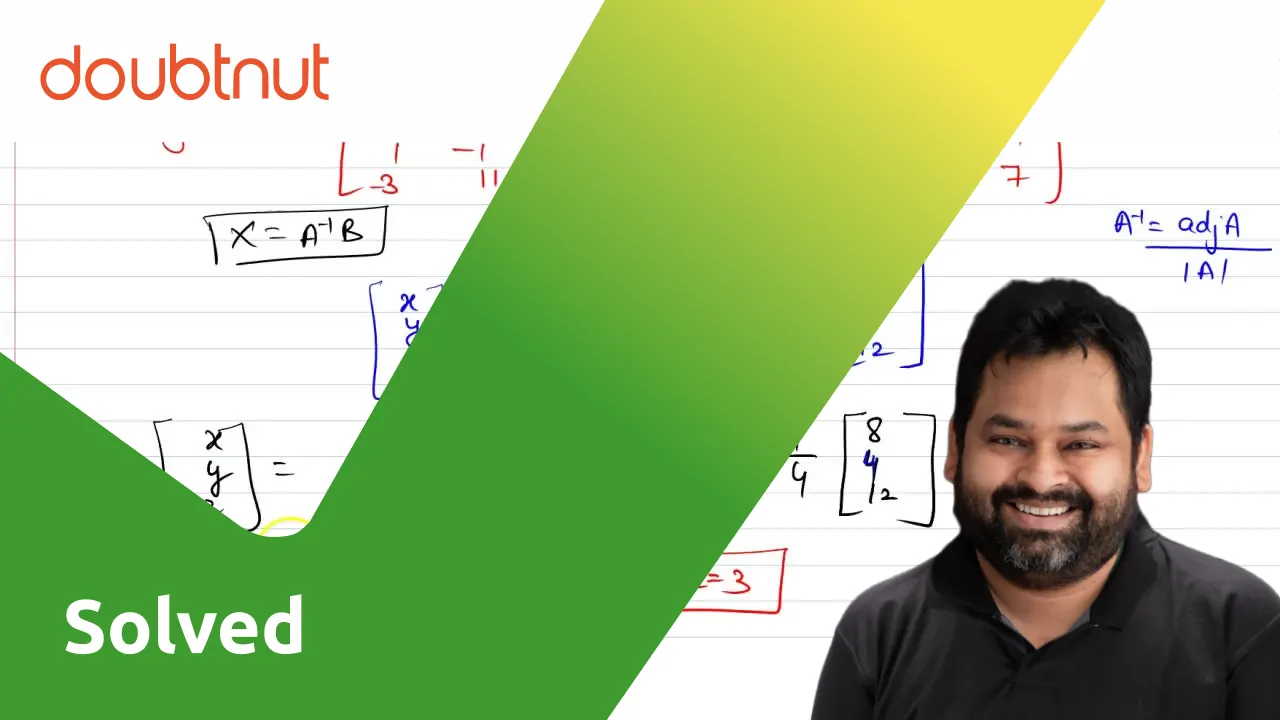Doubtnut App - Get 50 Lakh+ video solutions better than GoogleInstall Now

# Using matrices, solve the following system of linear equations: x − y+2z=7 3x+4y − 5z=−5 2x − y+3z=12

Video Solution